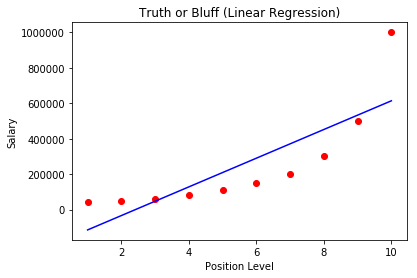• +91-9872993883
• +91-8283824812
• info@ris-ai.com

# Prediction Of Employee Salary On The Bases Of Previous Company Data With Polynomial Regression ¶

Project Objective: Lets assume the HR team of a company uses to determine what salary to offer to a new employee. For our project, let's take an example that an employee has applied for the role of a Regional Manager and has already worked as a Regional Manager for 2 years. So based on the data provided(Position_Salaries.csv) from employee last company - he falls between level 6 and level 7 - Lets say he falls under level 6.5. So, we want to build a model to predict what salary we should offer new employee if we come to know the true salary from previous company.

## Importing the libraries ¶

Firstly, we import necessary library(numpy, matplotlib and pandas) for this model.

In :
import numpy as np
import matplotlib.pyplot as plt
import pandas as pd


### Importing the dataset ¶

we need to predict the salary for an employee who falls under Level 6.5. So we really do not need the first column "Position". Here X is our independent variable which is the "Level" and y is the dependent variable which is the "Salary"

In :
dataset = pd.read_csv('Position_Salaries.csv')
print(dataset)   # Show all the data in Position_Salaries.csv file
X = dataset.iloc[:, 1:-1].values  #which simply means take all rows and all columns from index 1 upto index 2 but not including index 2
print("level", X)
y = dataset.iloc[:, -1].values  #which simply means take all rows and only columns with index 2
print("salary", y)

            Position  Level   Salary
1  Junior Consultant      2    50000
2  Senior Consultant      3    60000
3            Manager      4    80000
4    Country Manager      5   110000
5     Region Manager      6   150000
6            Partner      7   200000
7     Senior Partner      8   300000
8            C-level      9   500000
9                CEO     10  1000000
level [[ 1]
[ 2]
[ 3]
[ 4]
[ 5]
[ 6]
[ 7]
[ 8]
[ 9]
]
salary [  45000   50000   60000   80000  110000  150000  200000  300000  500000
1000000]


#### Fit Linear Regression model to dataset ¶

First we will build a simple linear regression model to see what prediction it makes and then compare it to the prediction made by the Polynomial Regression to see which is more accurate.

We will be using the LinearRegression class from the library sklearn.linear_model. We create an object of the LinearRegression class and call the fit method passing the X and y.

In :
# Training the Linear Regression model on the whole dataset
from sklearn.linear_model import LinearRegression
lin_reg = LinearRegression()
lin_reg.fit(X, y)

Out:
LinearRegression(copy_X=True, fit_intercept=True, n_jobs=None, normalize=False)

# Salary Prediction of an Employee¶

#### Visualization of linear regression¶

Lets plot the graph to look at the results for Linear Regression

In :
# Visualising the Linear Regression results
plt.scatter(X, y, color = 'red')
plt.plot(X, lin_reg.predict(X), color = 'blue')
plt.title('Truth or Bluff (Linear Regression)')
plt.xlabel('Position Level')
plt.ylabel('Salary')
plt.show()#### Training the Polynomial Regression model on the whole dataset ¶

In :
from sklearn.preprocessing import PolynomialFeatures
poly_reg = PolynomialFeatures(degree = 4)
X_poly = poly_reg.fit_transform(X)
lin_reg_2 = LinearRegression()
lin_reg_2.fit(X_poly, y)

Out:
LinearRegression(copy_X=True, fit_intercept=True, n_jobs=None, normalize=False)

#### Convert X to Polynomial Format¶

We will be using the PolynomialFeatures class from the sklearn.preprocessing library for this purpose. When we create an object of this class - we have to pass the degree parameter. Lets begin by choose degree as 4 for more accuracy. Then we call the fit_transform method to transform matrix X.

In :
from sklearn.preprocessing import PolynomialFeatures
poly_reg = PolynomialFeatures(degree=4)
X_poly = poly_reg.fit_transform(X)


#### Fitting Polynomial Regression¶

Now we will create a new linear regression object called lin_reg_2 and pass X_poly to it instead of X.

In :
in_reg_2 = LinearRegression()
lin_reg_2.fit(X_poly,y)

Out:
LinearRegression(copy_X=True, fit_intercept=True, n_jobs=None, normalize=False)

#### Visualize Polynomial Regression Results ¶

Lets plot the graph to look at the results for Polynomial Regression

In :
plt.scatter(X,y, color="red")
plt.plot(X, lin_reg_2.predict(poly_reg.fit_transform(X)))
plt.title("Poly Regression Degree 2")
plt.xlabel("Level")
plt.ylabel("Salary")
plt.show()If we look at the graph, we can see that a person at level 6.5 should be offered a salary of around $190k. We will confirm this in next step. #### Predict Polynomial Regression Results¶ In : lin_reg_2.predict(poly_reg.fit_transform([[6.5]]))  Out: array([158862.45265158]) We get a prediction of$158k which looks reasonable based on our dataset

So in this case by using Linear Regression - we got a prediction of \$330k and by using Polynomial Regression we got a prediction of 158k. which is shows that Polynomial Regression is mor reasonable.